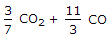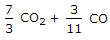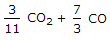# Mechanical Engineering - Thermodynamics - Discussion

### Discussion :: Thermodynamics - Section 6 (Q.No.4)

4.

The mass of carbon per kg of flue gas is given by

 [A].[B].[C].[D].Explanation:

No answer description available for this question.

 Harvinder Singh said: (Oct 6, 2014) Right answer is 11/3 CO2 and 7/3 CO.

 Imran Ali said: (Nov 14, 2014) A is right answer the mass of carbon per kg of dry flue gas is given by 11/3 CO2 and 3/7 CO.

 Siva Bharath said: (Jul 3, 2015) I didn't get the answer. Please if you know well then explain.

 Grandhi Sandeep said: (Aug 2, 2015) Some amendments need to be done for the options.

 Kaushal said: (Jan 30, 2016) What is flue gas?

 Sandeep Das said: (May 6, 2016) Can anyone explain the solution?

 Biren Desai said: (Jul 8, 2016) Answer is wrong right answer is 3/11 co2 and 3/7 Co.

 Rayudu said: (Jul 14, 2016) Flue gas is the gas exiting to the atmosphere via a flue, which is a pipe or channel for conveying exhaust gases from a fireplace, oven, furnace, boiler or steam generator. Quite often, the flue gas refers to the combustion exhaust gas produced at power plants.

 Daka Hitesh said: (Aug 23, 2016) The right answer is 3/11 CO2 and 3/7 CO.

 Suresh Kamal said: (Aug 29, 2016) Please explain step by step.

 Sarfaraz said: (Dec 14, 2016) Mass of carbon = 12 Mass of O2 = 32. CO2(12+32) =44 + CO (12+16) = 28. Mass of carbon present 44/12 CO2 + 28/12 CO. 11/3 CO2 + 7/3 CO.

 Biplab said: (Dec 28, 2016) CO2 + CO = C. C = 12, O2 = 32, O = 16, CO2 = 32 + 12 = 44, CO = 12 + 16 = 28, CO2 + CO = C, 44 kg + 28 kg =12 kg, 44/12 kg + 28/12 =1 kg carbon, 11/3 kg + 7/3 kg = 1 kg carbon.

 Durgesh Kumar Dubey said: (Jan 4, 2017) We know that one kg of carbon produced 11/3 kg of CO2 and 7/3 kg of CO. So per kg of this gaseous, carbon will be produced 3/11 kg of CO2 and 3/7 kg of CO. So, according to me, no any answer is correct in above mention option.

 Anurup said: (Jun 15, 2017) It think 3/11CO2 + 3/7CO.

 Chahat Sharma said: (Jun 17, 2017) Yes, you are correct @Sarfaraz.

 Mehul said: (Dec 4, 2017) Right answer is 3/11CO2 + 3/7CO.

 J.T.R said: (Dec 9, 2017) No, the correct answer is 3/11 co2+ 3/7 co.

 K K said: (Feb 14, 2018) @Biplab. CO2 + CO = C is not balanced. I think Balanced equation is CO2+2CO=3C+2O2.

 Syed Sameer said: (Jul 10, 2018) The mass of carbon, contained in 1 kg of flue or exhaust gases, may be calculated from the mass of carbon dioxide and carbon monoxide present in them. We know that 1 kg of carbon produces 11/3 kg of carbon dioxide. Hence 1 kg of carbon dioxide will contain 3/11 kg of carbon. Also, 1 kg of carbon produces 7/3 kg of carbon monoxide, hence 1 kg of carbon monoxide will contain 3/7 kg of carbon. Mass of carbon per kg of flue gas is given by: 3/11CO2 + 3/7CO.

 Tejaa said: (Oct 21, 2018) Yes, right @Syed Sameer.

 Kapil said: (Aug 12, 2019) I think the answer would be 3/11CO2 + 3/7CO.

 Velmurugan R said: (Aug 27, 2019) The mass of carbon, contained in 1 kg of flue or exhaust gases, may be calculated from the mass of carbon dioxide and carbon monoxide present in them. We know that 1 kg of carbon produces 11 / 3 kg of carbon dioxide. Hence 1 kg of carbon dioxide will contain 3 / 11 kg of carbon. Also, 1 kg of carbon produces 7/3 kg of carbon monoxide, hence 1 kg of carbon monoxide will contain 3/7 kg of carbon. Mass of carbon per kg of flue gas is given by: 3/11 CO2+ 3/7 CO.